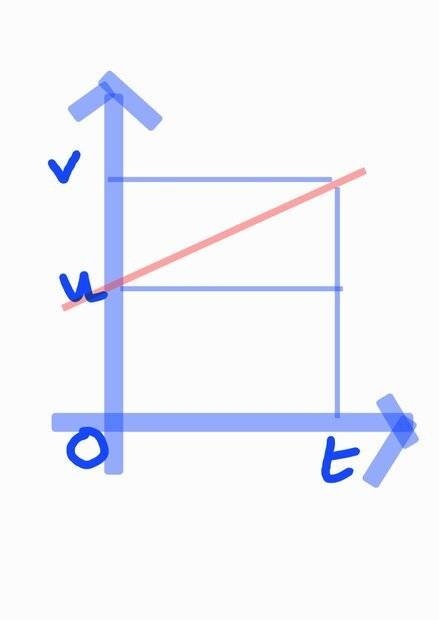# Revisiting Average Velocity

• B
• agnimusayoti

#### agnimusayoti

I read Tipler's book: Physics for Scientists and Engineer and he derived the equation of motion for linear motion with constant acceleration algebraic. He stated that for linear motion with constant acceleration, the average velocity can be determined with mean value of initial and final velocity.

I wonder why we can take the mean value of only those 2 velocity? Thank you.

That's how the maths falls out. Note that the average velocity is the total distance traveled divided by the time taken.

Algebraically, you can write down a formula for distance traveled in terms of the initial speed ##u##, the acceleration ##a##, and the time taken ##t##. And you can write down an expression for the final velocity ##v## in terms of the same quantities. You can then eliminate ##a## from the first expression and divide by ##t## to get the average velocity. With a bit of algebra, ##(v-u)/2## should drop out.

Graphically, plot velocity as a function of time. It should look like the red line:(Forgive the unlabelled axes - the horizontal one is time and the vertical is velocity.) Distance traveled is the area under the line on this kind of graph (because it's ##\int v(t)dt##). The average velocity is the constant velocity you would have to travel at to cover the same distance in the same time. Where would you have to draw a horizontal line (i.e. constant velocity) so that the area under the graph between times 0 and ##t## is the same? (Hint: the area of a triangle is half base times height). Does your answer depend on anything except ##u## and ##v##?

•agnimusayoti and Lnewqban